# Excel 神器 —— OpenPyXl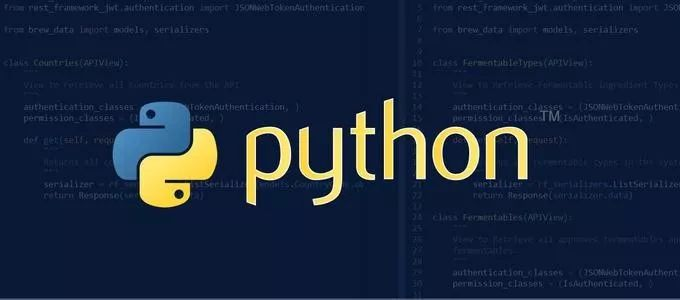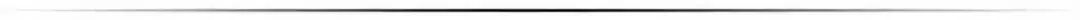## Python 下的 Excel 库

Python 中有大量的原生和第三方 Excel 操作包，各有所长，不过对于刚使用 Python 与 Excel 交互的同学来说，可能有点目不暇接，所以先简单梳理一下常见的一些 Excel 包

• OpenPyXL 是个读写 Excel 2010 xlsx/xlsm/xltx/xltm 的 Python 库，简单易用，功能广泛，单元格格式/图片/表格/公式/筛选/批注/文件保护等等功能应有尽有，图表功能是其一大亮点

• xlwings 是一个基于 BSD 授权协议的 Python 库，可以轻松的使用 Python 操作 Excel，也可以在 Excel 中调用 Python，以接近 VBA 语法的实现 Excel 编程，支持 Excel 宏，并且可以作为 Web 服务器，提供 REST API 接口

• pandas 数据处理是 pandas 的立身之本，Excel 作为 pandas 输入/输出数据的容器

• win32com 从命名上就可以看出，这是一个处理 windows 应用的扩展，Excel 只是该库能实现的一小部分功能。该库还支持 office 的众多操作。需要注意的是，该库不单独存在，可通过安装 pypiwin32 或者 pywin32 获取

• Xlsxwriter 拥有丰富的特性，支持图片/表格/图表/筛选/格式/公式等，功能与 openpyxl 相似，优点是相比 openpyxl 还支持 VBA 文件导入，迷你图等功能，缺点是不能打开/修改已有文件，意味着使用 xlsxwriter 需要从零开始

• DataNitro 一个 Excel 的付费插件，内嵌到 Excel 中，可完全替代 VBA，在 Excel 中使用 python 脚本。既然被称为 Excel 中的 python，同时可以与其他 python 库协同。

• xlutils 基于 xlrd/xlwt，老牌 python 包，算是该领域的先驱，功能特点中规中矩，比较大的缺点是仅支持 xls 文件。

• 不想使用 GUI 而又希望赋予 Excel 更多的功能，openpyxl 与 xlsxwriter，二者可选其一；

• 需要进行科学计算，处理大量数据，建议 pandas+xlsxwriter 或者 pandas + openpyxl，是不错的选择；

• 想要写 Excel 脚本，会 Python 但不会 VBA，可考虑 xlwings 或 DataNitro；

• win32com 功能还是性能都很强大，不过需要一定的 windows 编程经验才能上手，它相当于是 windows COM 的封装，另外文档不够完善

## OpenPyXL

OpenPyXl 几乎可以实现所有的 Excel 功能，而且接口清晰，文档丰富，学习成本相对较低，今天就以 OpenPyXL 为例，了解下如何操作 Excel

### 安装

pip install openpyxl


python -c "import openpyxl"


### 基本概念

• workbook 相当于一个 Excel 文件档，每个被创建和打开的 Excel 文件都是独立的 Workbook 对象

• sheet Excel 文档中的表单，每个 Excel 文档至少需要一个 sheet

• cell 单元格，是不可分割的基本数据存储单元

### 小试牛刀

from openpyxl import Workbook
# 创建一个 workbook
wb = Workbook()
# 获取被激活的 worksheet
ws = wb.active
# 设置单元格内容
ws['A1'] = 42
# 设置一行内容
ws.append([1, 2, 3])
# python 数据类型可以被自动转换
import datetime
ws['A2'] = datetime.datetime.now()
# 保存 Excel 文件
wb.save("sample.xlsx")


• 新创建的 workbook 对象，会自带一个名为 Sheet 的表单，Office Excel 新建会创建 3 个

• 创建的 workbook 会将第一个 表单 激活，通过 wb.active 获取引用

• 像 python-docx work 库一样，save 方法会立即保存，不会有任何提示，建议选择不同文件名来保存

## 常用功能

OpenPyXl 功能很多，从单元格处理到图表展示，涵盖了几乎全部的 Excel 功能，这里就一些常用的功能做展示，更多的用法可以参考 OpenPyXl 文档（文末参考里有链接）

### 创建和打开 Excel

from openpyxl import load_workbook

# 显示文档中包含的 表单 名称
print(wb.sheetnames)


load_workbook 除了参数 filename外为还有一些有用的参数：

• read_only：是否为只读模式，对于超大型文件，要提升效率有帮助

• keep_vba ：是否保留 vba 代码，即打开 Excel 文件时，开启并保留宏

• guess_types：是否做在读取单元格数据类型时，做类型判断

• data_only：是否将公式转换为结果，即包含公式的单元格，是否显示最近的计算结果

• keep_links：是否保留外部链接

### 操作 sheet

from openpyxl import Workbook
wb = Workbook()
ws = wb.active

ws1 = wb.create_sheet("sheet")  #创建一个 sheet 名为 sheet
ws1.title = "新表单"  # 设置 sheet 标题
ws2 = wb.create_sheet("mysheet", 0) # 创建一个 sheet，插入到最前面 默认插在后面
ws2.title = u"你好"  # 设置 sheet 标题

ws1.sheet_properties.tabColor = "1072BA"  # 设置 sheet 标签背景色

# 获取 sheet
ws3 = wb.get_sheet_by_name(u"你好")
ws4 = wb['New Title']

# 复制 sheet
ws1_copy = wb.copy_worksheet(ws1)

# 删除 sheet
wb.remove(ws1)

• 每个 Workbook 中都有一个被激活的 sheet，一般都是第一个，可以通过 active 直接获取

• 可以通过 sheet 名来获取 sheet 对象

• 创建 sheet时需要提供 sheet 名称参数，如果该名称的 sheet 已经存在，则会在名称后添加 1，再有重复添加 2，以此类推

• 获得 sheet 对象后，可以设置 名称（title），背景色等属性

• 同一个 Workbook 对象中，可以复制 sheet，需要将源 sheet 对象作为参数，复制的新 sheet 会在最末尾

• 可以删除一个 sheet，参数是目标 sheet 对象

### 操作单元格

OpenPyXl 可以操作单个单元格，也可以批量操作单元格

#### 单独操作

ws1 = wb.create_sheet("Mysheet")  #创建一个sheet
# 通过单元格名称设置
ws1["A1"]=123.11
ws1["B2"]="你好"

# 通过行列坐标设置
d = ws1.cell(row=4, column=2, value=10)

• 可以通过单元格名称设置，类似于 sheet 的某种属性

• 也可以通过行列坐标类设置

#### 批量操作

• 指定行列

# 操作单列
for cell in ws["A"]:
print(cell.value)
# 操作单行
for cell in ws["1"]:
print(cell.value)
# 操作多列
for column in ws['A:C']:
for cell in column:
print(cell.value)
# 操作多行
for row in ws['1:3']:
for cell in row:
print(cell.value)
# 指定范围
for row in ws['A1:C3']:
for cell in row:
print(cell.value)

• 所有行或者列

# 所有行
for row in ws.iter_rows():
for cell in row:
print(cell.value)
# 所有列
for column in ws.iter_cols():
for cell in column:
print(cell.value)

• 设置整行数据

ws.append((1,2,3))


#### 合并单元格

# 合并
ws.merge_cells('A2:D2')
# 解除合并
ws.unmerge_cells('A2:D2')

ws.merge_cells(start_row=2,start_column=1,end_row=2,end_column=4)
ws.unmerge_cells(start_row=2,start_column=1,end_row=2,end_column=4)

• sheet 对象的 merge_cells 方法是合并单元格，unmerge_cells 是解除合并

• 分别有两种参数形式，一种是用单元格名称方式指定，另一种是通过命名参数指定

• 注意：对于没有合并过单元格的位置调用 unmerge_cells 时会报错

### 单元格格式

OpenPyXl 用6种类来设置单元格的样式

• NumberFormat 数字

• Alignment 对齐

• Font 字体

• Border 边框

• PatternFill 填充

• Protection 保护

from openpyxl.styles import Font, PatternFill, Border, Side, Alignment, Protection
from openpyxl.styles import numbers

wb = Workbook()
ws = wb.active
ws.cell(row=1, column=1, value='宋体').font = Font(name=u'宋体', size=12, bold=True, color='FF0000')
ws.cell(row=2, column=2, value='右对齐').alignment = Alignment(horizontal='right')
ws.cell(row=3, column=3, value='填充渐变色').fill = PatternFill(fill_type='solid', start_color='FF0000')
ws.cell(row=4, column=4, value='设置边线').border = Border(left=Side(border_style='thin', color='FF0000'), right= Side(border_style='thin', color='FF0000'))
ws.cell(row=5, column=5, value='受保护的').protection = Protection(locked=True, hidden=True)
ws.cell(row=6, column=6, value=0.54).number_format =numbers.FORMAT_PERCENTAGE

• 引入字体类

• 用 cell 方法，为单元格设置值的同时，设置格式

• 每种格式都有特定的属性，为其设置特定的格式对象

• 数字格式有点区别，通过设置格式名称来完成，numbers.FORMAT_PERCENTAGE 是个字符串

• Border 类，需要配合 Side 类使用，它们都在 openpyxl.styles 中定义

• 需要注意的是，单元格样式属性只能通过样式对象赋予，而无法通过样式属性来修改，例如 ws.cell(1, 1).font.color = '00FF00' 会报错，如果真要换，需要重新创建一个样式实体，重新赋值

font = Font(bold=True)

# 遍历范围内的单元格
for row in ws['A1:C3']:
for cell in row:
cell.font = font

# 设置整行
row = ws.row_dimensions
row.font = font

# 设置整列
column = ws.column_dimensions["A"]
column.font = font


### 图表

#### 柱状图

from openpyxl import Workbook
from openpyxl.chart import BarChart, Reference

wb = Workbook()
ws = wb.active

rows = [
('月份', '苹果', '香蕉'),
(1, 43, 25),
(2, 10, 30),
(3, 40, 60),
(4, 50, 70),
(5, 20, 10),
(6, 10, 40),
(7, 50, 30),
]

for row in rows:
ws.append(row)

chart1 = BarChart()
chart1.type = "col"
chart1.style = 10
chart1.title = "销量柱状图"
chart1.y_axis.title = '销量'
chart1.x_axis.title = '月份'

data = Reference(ws, min_col=2, min_row=1, max_row=8, max_col=3)
series = Reference(ws, min_col=1, min_row=2, max_row=8)
chart1.set_categories(series)

• 引入柱状图类 BarChart 和 数据应用类 Reference

• 创建 Workbook，并为活动 Sheet 添加数据

• 创建柱状图对象，设置图表属性，type 为 col 为列状图，bar 为水平图

• 创建数据引用对象，指定从那个 sheet 以及数据范围

• 创建系列数据引用对象

• 将数据和系列加入到图表对象中

• 最后将图表对象用 add_chart 添加到 sheet 里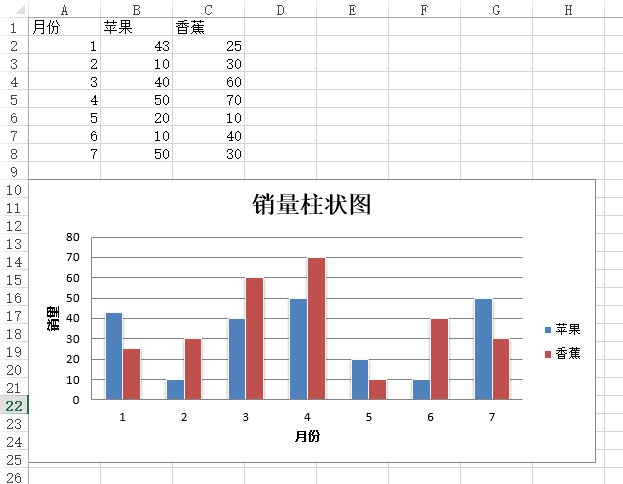#### 圆饼图

from openpyxl import Workbook
from openpyxl.chart import PieChart, Reference

data = [
['水果', '销量'],
['苹果', 50],
['樱桃', 30],
['橘子', 10],
['香蕉', 40],
]

wb = Workbook()
ws = wb.active

for row in data:
ws.append(row)

pie = PieChart()
pie.title = "水果销量占比"
labels = Reference(ws, min_col=1, min_row=2, max_row=5)
data = Reference(ws, min_col=2, min_row=1, max_row=5)
pie.set_categories(labels)


• 引入饼图类 PieChart 和 数据应用类 Reference

• 创建图表数据

• 创建图表对象，设置图表标题

• 定义标签数据引用和数据引用，并将其加入到图表

• 将图表对象添加到 sheet 的指定位置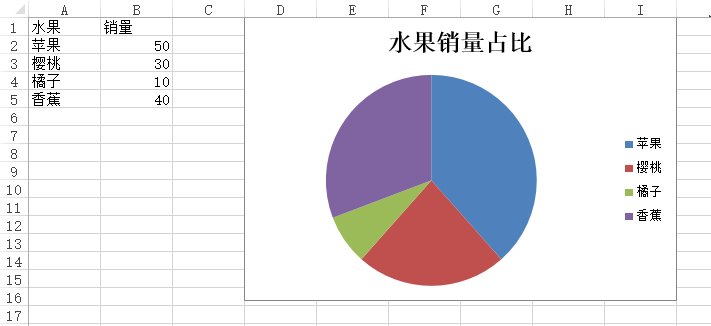## 参考

• Excel 作画 https://zhuanlan.zhihu.com/p/34917620

• https://www.jianshu.com/p/be1ed0c5218e

• https://www.douban.com/note/706513912/

• https://blog.csdn.net/weixin_41595432/article/details/79349995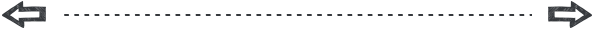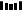11-0631608-28270
02-142504
07-048955
07-122万+
07-191万+
01-03537
12-111363
07-19373
07-14622点击重新获取扫码支付余额充值